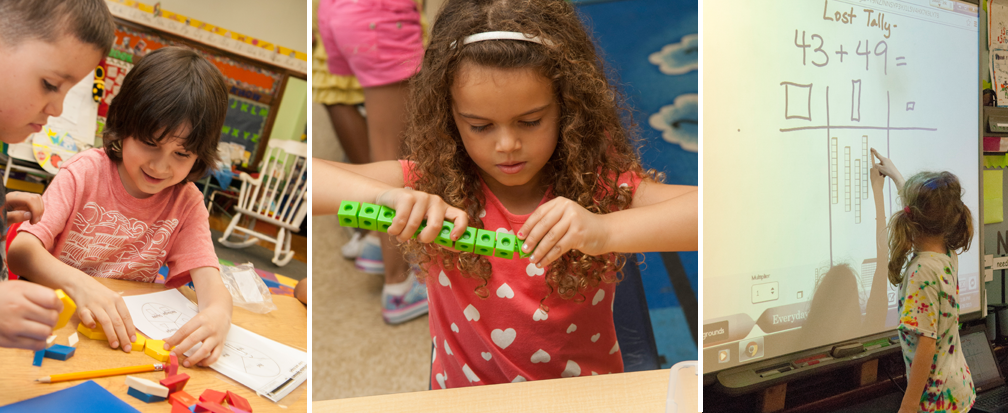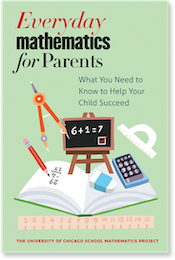Everyday Mathematics third-edition Grade Level Goals have been revised to align with the Common Core State Standards for Mathematics (CCSS-M), which were widely adopted in 2010. Both versions of the curriculum's goals are available below. For more information on standards, please see Meeting Standards with Everyday Mathematics.

### Common Core State Standards (CCSS) Edition Grade Level Goals

Expand All | Collapse All
Number and Numeration
Understand the Meanings, Uses, and Representations of Numbers Rote counting Count on by 1s, 2s, 5s, and 10s past 100 and back by 1s from any number less than 100 with and without number grids, number lines, and calculators.
Rational counting Count collections of objects accurately and reliably; estimate the number of objects in a collection.
Place value and notation Read, write, and model with manipulatives whole numbers up to 1,000; identify places in such numbers and the values of the digits in those places.
Meanings and uses of fractions Use manipulatives and drawings to model halves, thirds, and fourths as equal parts of a region or a collection; describe the model.
Number theory Use manipulatives to identify and model odd and even numbers.
Understand Equivalent Names for Numbers Equivalent names for whole numbers Use manipulatives, drawings, tally marks, and numerical expressions involving addition and subtraction of 1- or 2-digit numbers to give equivalent names for whole numbers up to 100.
Understand Common Numerical Relations Comparing and ordering numbers Compare and order whole numbers up to 1,000.
Operations and Computation
Compute Accurately Addition and subtraction facts Demonstrate appropriate fluency with addition and subtraction facts through 10+10.
Addition and subtraction procedures Use manipulatives, number grids, tally marks, mental arithmetic, and calculators to solve problems involving the addition and subtraction of 1-digit whole numbers with 2-digit whole numbers; calculate and compare the values of combinations of coins.
Make Reasonable Estimates Computational estimation Estimate reasonableness of answers to basic fact problems (e.g., Will 7+8 be more or less than 10?).
Understand Meanings of Operations Models for the operations Identify change-to-more, change-to-less, comparison, and parts-and-total situations.
Data and Chance
Select and Create Appropriate Graphical Representations of Collected or Given Data Data collection and representation Collect and organize data to create class-constructed tally charts, tables, bar graphs, and line plots.
Analyze and Interpret Data Data analysis Use graphs to answer simple questions and draw conclusions; find the maximum and minimum of a data set.
Understand and Apply Basic Concepts of Probability Qualitative probability Describe events using certain, likely, unlikely, impossible, and other basic probability terms.
Measurement and Reference Frames
Understand the Systems and Processes of Measurement; Use Appropriate Techniques, Tools, Units, and Formulas in Making Measurements Length, weight, and angles Use nonstandard tools and techniques to estimate and compare weight and length; measure length with standard measuring tools.
Money Know and compare the value of pennies, nickels, dimes, quarters, and dollar bills; make exchanges between coins.
Use and Understand Reference Frames Temperature Identify a thermometer as a tool for measuring temperature; read temperatures on Fahrenheit and Celsius thermometers to the nearest 10Â¯.
Time Use a calendar to identify days, weeks, months, and dates; tell and show time to the nearest half and quarter hour on an analog clock.
Geometry
Investigate Characteristics and Properties of Two- and Three-Dimensional Geometric Shapes Plane and solid figures Identify and describe plane and solid figures including circles, triangles, squares, rectangles, spheres, cylinders, rectangular prisms, pyramids, cones, and cubes.
Apply Transformations and Symmetry in Geometric Situations Transformations and symmetry Identify shapes having line symmetry; complete line-symmetric shapes or designs.
Patterns, Functions, and Algebra
Understand Patterns and Functions Patterns and functions Extend, describe, and create numeric, visual, and concrete patterns; solve problems involving function machines, "What_s My Rule?" tables, and Frames-and-Arrows diagrams.
Use Algebraic Notation to Represent and Analyze Situations and Structures Algebraic notation and solving number sentences Read, write, and explain expressions and number sentences using the symbols +,-, and = and the symbols > and < with cues; solve equations involving addition and subtraction.
Properties of the arithmetic operations Apply the Commutative and Associative Properties of Addition and the Additive Identity to basic addition fact problems.

### 3rd Edition Grade Level Goals

Expand All | Collapse All
Number and Numeration
Understand the Meanings, Uses, and Representations of Numbers Rote counting Count on by 1s to 100; count on by 2s, 5s, and 10s and count back by 1s with number grids, number lines, and calculators.
Rational counting Count collections of objects accurately and reliably; estimate the number of objects in a collection
Place value and notation Read, write, and model with manipulatives whole numbers up to 1,000; identify places in such numbers and the values of the digits in those places.
Meanings and uses of fractions Use manipulatives and drawings to model halves, thirds, and fourths as equal parts of a region or a collection; describe the model.
Number theory Use manipulatives to identify and model odd and even numbers
Understand Equivalent Names and Numbers Equivalent names for whole numbers Use manipulatives, drawings, tally marks, and numerical expressions involving addition and subtraction of 1- or 2-digit numbers to give equivalent names for whole numbers up to 100.
Understand Numerical Relations Comparing and ordering numbers Compare and order whole numbers up to 1,000.
Operations and Computation
Computes Accurately Addition and subtraction facts Demonstrate proficiency with +/-0, +/- 1, doubles, and sum-equals-ten addition and subtraction facts such as 6+4=10, and 10-7=3
Addition and subtraction procedures Use manipulatives, number grids, tally marks, mental arithmetic, and calculators to solve problems involving the addition and subtraction of 1-digit whole numbers; calculate and compare the values of combinations and coins
Make Reasonable Estimates Computational estimation Estimate reasonableness of answers to basic fact problems (e.g., Will 7+8 be more or less than 10?).
Understand Meanings of Operations Models for the operations Identify change to more, change-to-less, comparison, and parts-and-total situations.
Data and Chance
Select and Create Appropriate Graphical Representations of Collected or Given Data Data collection and representation Collect and organize data to create class-constructed tally charts, tables, bar graphs, and line plots.
Analyze and Interpret Data Data analysis Use graphs to answer simple questions and draw conclusions; find the maximum and minimum of a data set.
Understand and Apply Basic Concepts of Probability Qualitative probability Describe events using certain, likely, unlikely, impossible, and other basic probability terms.
Measurement and Reference Frames
Understand the Systems and Processes of Measurement; Use Appropriate Techniques, Tools, Units, and Formulas in Making Measurements Length, weight, and angles Use nonstandard tools and techniques to estimate and compare weight and length; measure length with standard measuring tools.
Money Know and compare the value of pennies, nickels, dimes, quarters, and dollar bills; make exchanges between coins.
Use and Understand Reference Frames Temperature Identify a thermometer as a tool for measuring temperature; read temperatures on Fahrenheit and Celsius thermometers to the nearest 10°.
Time Use a calculator to identify the days, weeks, months, and dates; tell and show time to the nearest half and quarter on an analog clock.
Geometry
Investigate Characteristics and Properties of Two- and Three-Dimensional Geometric Shapes Plane and solid figures Identify and describe plane and solid figures including circles, triangles, squares, rectangles, spheres, cylinders, rectangular prisms, pyramids, cones, and cubes.
Apply Transformations and Symmetry in Geometric Situations Transformations and symmetry Identify shapes having line symmetry; complete line-symmetric shapes or designs.
Patterns, Functions, and Algebra
Understand Patterns and Functions Patterns and functions Extend, describe, and create numeric, visual, and concrete patterns; solve problems involving function machines, "What’s My Rule?" tables, and Frames-and Arrows diagrams..
Use Algebraic Notation to Represent and Analyze Situations and Structures Algebraic notation and solving number sentences Read, write, and explain expressions and number sentences using the symbols +,-, and = and the symbols > and < with cues; solve equations involving addition and subtraction.
Properties of arithmetic operations Apply the Commutative Property of Addition and the Additive Identity to basic addition fact problems.Everyday Mathematics for Parents: What You Need to Know to Help Your Child Succeed

The University of Chicago School Mathematics Project

University of Chicago Press